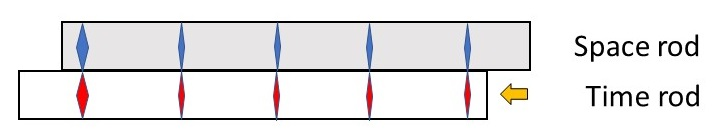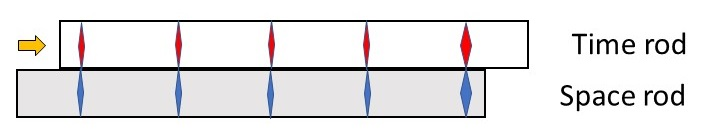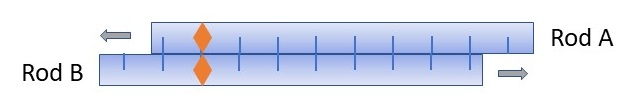# Rod-clocks and clock-rods

Consider an analogue clock with a dial and a hand, i.e., a pointer, reimagined as two circular bands:The Dial band is at rest relative to the observer and the Hand band turns clockwise at a fixed rate. The pointer on the Hand band points to the marking on the Dial band that indicates the time. In an equivalent but opposite configuration the Hand band is at rest relative to the observer and the Dial band turns counterclockwise at the same fixed rate.

Next unroll these two bands into two rods.The Hand rod moves uniformly to the right along the Dial rod, which is at rest relative to the observer. The pointer on the Hand rod points to the mark on the Dial rod, which indicates the time, that is, the duration since pointing to zero. This is a rod-clock, i.e., a clock made from rods.

Then make the two rods similar by marking both of them with numerals and pointers, for example like this:

One advantage is that duration can be measured at any point of the Dial as the difference between two pointers on the Hand rod. Note that the scale of the two rods can be different, though for simplicity they would be the same.

In an equivalent but opposite configuration, the Dial rod moves uniformly to the left along the Hand rod, which is at rest relative to the observer. This is a clock-rod, i.e., a rod which is clock-like. One advantage is that length can be measured at any point of the Hand adjacent to a mark on the Dial rod.Length is measured with rods as the difference between the values indicated by the pointer at two places. If the rods’ relative motion is uniform, then the length and duration measured is proportional, the only difference being the units on the rod. Compare answering the question, “how far away” with the distance or the typical travel time.